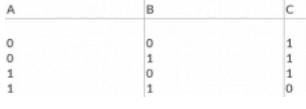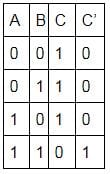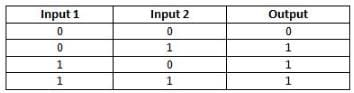NEET  >  Test: Logic Gates

# Test: Logic Gates

Test Description

## 10 Questions MCQ Test Physics Class 12 | Test: Logic Gates

Test: Logic Gates for NEET 2023 is part of Physics Class 12 preparation. The Test: Logic Gates questions and answers have been prepared according to the NEET exam syllabus.The Test: Logic Gates MCQs are made for NEET 2023 Exam. Find important definitions, questions, notes, meanings, examples, exercises, MCQs and online tests for Test: Logic Gates below.
Solutions of Test: Logic Gates questions in English are available as part of our Physics Class 12 for NEET & Test: Logic Gates solutions in Hindi for Physics Class 12 course. Download more important topics, notes, lectures and mock test series for NEET Exam by signing up for free. Attempt Test: Logic Gates | 10 questions in 10 minutes | Mock test for NEET preparation | Free important questions MCQ to study Physics Class 12 for NEET Exam | Download free PDF with solutions
 1 Crore+ students have signed up on EduRev. Have you?
Test: Logic Gates - Question 1

### The binary number system has a base of​

Detailed Solution for Test: Logic Gates - Question 1

Binary number system, in mathematics, positional numeral system employing 2 as the base and so requiring only two different symbols for its digits, 0 and 1, instead of the usual 10 different symbols needed in the decimal system.

Test: Logic Gates - Question 2

### In the binary number 10010, the MSB is​

Detailed Solution for Test: Logic Gates - Question 2

1001=1x23+0x22+0x21+1x20=9
For binary numbers, the digit at the extreme right is referred to as least significant bit (LSB) and the left- most digit is called the most significant bit (MSB). Hence in binary 10010, the most significant bit is 1.

Test: Logic Gates - Question 3

### Which of the following is the universal gate?

Detailed Solution for Test: Logic Gates - Question 3

A universal gate is a gate which can implement any Boolean function without need to use any other gate type. The NAND and NOR gates are universal gates. In practice, this is advantageous since NAND and NOR gates are economical and easier to fabricate and are the basic gates used in all IC digital logic families.

Test: Logic Gates - Question 4

The inputs of a NAND gate are connected together. The resultant circuit is

Detailed Solution for Test: Logic Gates - Question 4

The equation of NAND gate is
Y=Aˉ.Bˉ
When both the terminals are connected together.
A=B=A
Y=Aˉ.Aˉ=Aˉ.Aˉ=Aˉ
Which is nothing but the equation of NOT gate.
Y=Aˉ
Option A is the correct answer.

Test: Logic Gates - Question 5

The NOR gate is OR gate followed by

Detailed Solution for Test: Logic Gates - Question 5

An OR gate followed by a NOT gate in a cascade is called a NOR gate. In other words, the gate which provides a high output signal only when there are low signals on the inputs such type of gate is known as NOR gate.

Test: Logic Gates - Question 6

The truth table given below is for a gate with A and B as inputs and C as output. Which of the gates will obey this truth table?Detailed Solution for Test: Logic Gates - Question 6We can observe from the table that Yˉ=A.B⇒Y=AB
So, the table represents a NAND gate.

Test: Logic Gates - Question 7

Which among the following gates has output = A . B ?​

Detailed Solution for Test: Logic Gates - Question 7

The logic or Boolean expression given for a digital logic AND gate is that for Logical Multiplication which is denoted by a single dot or full stop symbol, ( . ) giving us the Boolean expression of:  A.B = Y.

Then we can define the operation of a digital 2-input logic AND gate as being:
“If both A and B are true, then Y is true”

Test: Logic Gates - Question 8

The output of a 2-input OR gate is zero only when its:

Detailed Solution for Test: Logic Gates - Question 8

The truth table for 2-input OR gate is as shown in the figure. Thus, the output is zero only when both inputs are zero.Test: Logic Gates - Question 9

If A = 1 and B = 0, what is the value of A.A+B?​

Detailed Solution for Test: Logic Gates - Question 9

A=1 and B=0
A.A+B
=A2+B [De-Morgan’s theorem)
=1+0
=1
=A

Test: Logic Gates - Question 10

The binary number 10101 is equivalent to decimal number

Detailed Solution for Test: Logic Gates - Question 10

10101 is the binary number to be converted to a decimal number.
To convert, multiply the numbers individually with 2n where n changes according to place value of the number.
(10101)2= (20× 1) + (0 × 21) + (1×22) + (0×23) + (1×24)
= 1 + 0 + 4 + 0 + 16
= 21

## Physics Class 12

157 videos|452 docs|213 tests
 Use Code STAYHOME200 and get INR 200 additional OFF Use Coupon Code
Information about Test: Logic Gates Page
In this test you can find the Exam questions for Test: Logic Gates solved & explained in the simplest way possible. Besides giving Questions and answers for Test: Logic Gates, EduRev gives you an ample number of Online tests for practice

## Physics Class 12

157 videos|452 docs|213 tests

### How to Prepare for NEET

Read our guide to prepare for NEET which is created by Toppers & the best Teachers